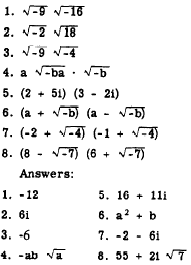Custom SearchADDITION AND SUBTRACTION OF COMPLEX NUMBERS Pure imaginaries are added and subtracted in the same way as any other algebraic quantities. The coefficients of similar terms are added or subtracted algebraically, as follows:Likewise, complex numbers in the rectangular form are combined like any other algebraic polynomials. Add or subtract the coefficients of similar terms algebraically. If parentheses enclose the numbers, first remove the parentheses. Next, place the real parts together and the imaginary parts together. Collect terms. As examples, consider the following: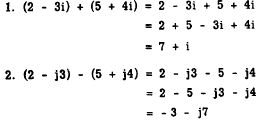In example 2, notice that the convention for writing operator j (the electronics form of the imaginary unit) with numerical coefficients is to place j first. If the complex numbers are placed one under the other, the results of addition and subtraction appear as follows:Practice problems. Add or subtract as indicated, in the following problems: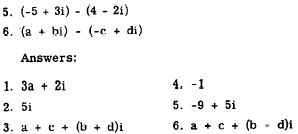MULTIPLICATION OF COMPLEX NUMBERS Generally, the rules for the multiplication of complex numbers and pure imaginaries are the same as for other algebraic quantities. However, there is one exception that should be noted: The rule for multiplying numbers under radical signs does not apply to TWO NEGATIVE numbers. When at least one of two radicands is positive, the radicands can be multiplied immediately, as in the following examples: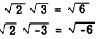When both radicands are negative, however, as in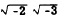, an inconsistent result is obtained if we multiply both numbers under the radical signs immediately. To get the correct result, express the imaginary numbers first in terms of i, as follows:Multiplying complex numbers is equivalent to multiplying binomials in the manner explained previously. After the multiplication is performed, simplify the powers of i as in the following examples: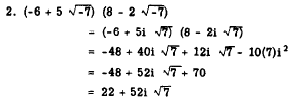Practice problems. Perform the indicated operations: